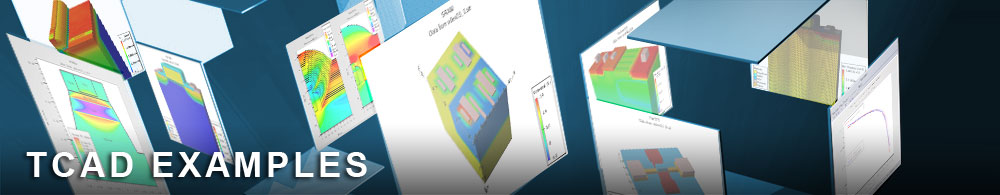Epitaxial GaAs MESFET S-Parameters

## mesfetex02.in : Epitaxial GaAs MESFET S-Parameters

Requires: Blaze
Minimum Versions: Atlas 5.28.1.R

This example demonstrates the calculation of s-parameters in a simple MESFET. It shows:

• MESFET structure definition using Atlas syntax
• Setting of GaAs mobility models and gate workfunction
• Id/Vds characteristics with Vgs=0
• AC analysis at a single DC bias at different frequencies
• Conversion of capacitance and conductance data from Atlas into s-parameters
• Smith Charts of MESFET s-parameters using TonyPlot

The geometry and doping of the MESFET device used in this example is described using the Atlas structural syntax. Initially the mesh is specified in x and y, using the following command format x.mesh loc=aaa spac=bbb specifying that there is a mesh spacing of bbb and x location aaa. region statements are used to specify two GaAs regions which will later be doped differently. electrode statements specify the names and positions of electrodes. The workfunction of the gate contact is set using contact . Each GaAs region is uniformly doped. An n-type active layer sits on top of a lowly doped p-type substrate. GaAs mobility models for concentration and field dependence are set in the models statement.

The numerical methods used at the initial stage of the simulation are conservative. The statement method gummel newton specifies that the decoupled (gummel) method is used at the start of the simulation for each bias point. This switches to the coupled (newton) method if convergence is not obtained. This is the most robust method for the initial bias steps and for complex devices. It does consume more CPU time and can lead to problems at high current levels. If these problems occur the statement method newton can be used.

The DC simulation proceeds by ramping the drain voltage in the solve statement until Vds=3.0V. After this, a log file is opened and AC analysis begins. The full sweep of frequency is done on the line:
solve ac.analysis direct frequency=1.e9 fstep=2.e9 nfsteps=20
The 'ac' parameter switches on the AC analysis. 'direct' specifies a robust AC solver for high frequencies. Activating the 'direct' solver will cause more memory to be allocated than was used during the DC simulation. If your system does not have enough virtual memory to run the AC application, the simulation will stop here with an error message. The exact amount of memory required depends on the number of mesh points. The 'frequency' value is the initial frequency of the AC signal, fstep is the step value added to this frequency, and nfsteps is the number of steps.

The AC analysis in Atlas calculates the real and imaginary current components from a small AC signal on top of the existing DC solution. From these currents Atlas calculates the conductance and capacitance between each pair of electrodes. By plotting the log file, users can see graphs of drain-gate conductance (C drain>gate) or total gate capacitance (-1*C gate>gate).

The s-parameters (or Z, Y and ABCD parameters) are calculated by setting the s.param (or y.param etc) on the log statement preceeding the ac analysis. The most important parameters are the *port parameters. s-parameter analysis assumes the device is a two port device with 4 terminals. The inport and outport are used to assign the respective electrodes. Users should ensure the correct definition of all ports. In this example the input port is set to the gate and output port to the drain.

To load and run this example, select the Load button in DeckBuild > Examples. This will copy the input file and any support files to your current working directory. Select the Run button in DeckBuild to execute the example.